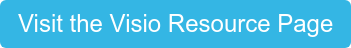At Visio Lending, our loan programs are designed to meet the needs of investors. That’s why when we determine a borrower’s ability to pay their mortgage, we look at the cash flow generated by the property, rather than the borrower’s personal income. To help us determine the monthly cash flow of a property, we use a ratio known as the Debt-Service Coverage Ratio or DSCR. DSCR is calculated by dividing the monthly rent by the monthly principal, interest, taxes, insurance, and association dues (PITIA).

So, what exactly are we looking for when we calculate this? A DSCR of 1.0 indicates the investor is breaking even; essentially the monthly rent that the borrower earns is the same as the monthly expenses. With a DSCR below a 1, the investor would be losing money, and a DSCR above a 1.2 is considered good. Let’s take a look at some examples.

## DSCR < 1

Principal + Interest= \$1,700

Taxes= \$350

Insurance= \$100

Association Dues=\$50

Total PITIA= \$2200

Rent= \$2000

DSCR= Rent/PITIA=2000/2200=0.91

Since the DSCR is .91, we know the expenses are greater than the income of the property.

## DSCR >1

Principal + Interest= \$1,500

Taxes= \$250

Insurance= \$100

Association Dues=\$25

Total PITIA= \$1875

Rent= \$2300

DSCR= Rent/PITIA=2300/1875=1.23

If we divide the rent by PITIA, we get a DSCR of 1.23, which indicates the property is cash flow positive.